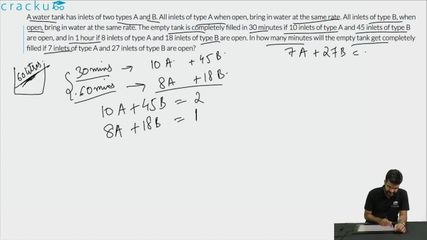Question 67

# A water tank has inlets of two types A and B. All inlets of type A when open, bring in water at the same rate. All inlets of type B, when open, bring in water at the same rate. The empty tank is completely filled in 30 minutes if 10 inlets of type A and 45 inlets of type B are open, and in 1 hour if 8 inlets of type A and 18 inlets of type B are open. In how many minutes will the empty tank get completely filled if 7 inlets of type A and 27 inlets of type B are open?

Solution

Let the efficiency of type A pipe be 'a' and the efficiency of type B be 'b'.
In the first case, 10 type A and 45 type B pipes fill the tank in 30 mins.
So, the capacity of the tank  = $$\dfrac{1}{2}$$(10a + 45b)........(i)
In the second case, 8 type A  and 18 type B pipes fill the tank in 1 hour.
So, the capacity of the tank = (8a + 18b)..........(ii)
Equating (i) and (ii), we get
10a + 45b = 16a + 36b
=>6a = 9b
From (ii), capacity of the tank = (8a + 18b) = (8a + 12a) = 20a
In the third case, 7 type A and 27 type B pipes fill the tank.
Net efficiency = (7a + 27b) = (7a + 18a) = 25a
Time taken = $$\dfrac{20\text{a}}{25\text{a}}$$ hour = 48 minutes.
Hence, 48 is the correct answer.

### View Video Solution